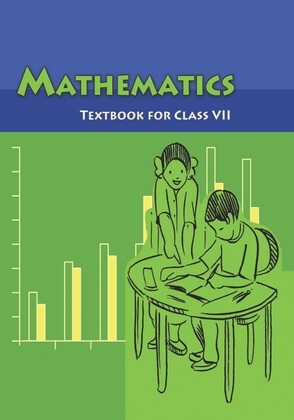•VISUALISING SOLID SHAPES EXERCISE 15.1 1. Identify the nets which can be used to make cubes (cut out copies of the nets and try it): (i) (ii) (iii) (iv) (v) (vi) 2. Dice are cubes with dots on each face. Opposite faces of a die always have a total of seven dots on them. Here are two nets to make dice (cubes); the numbers inserted in each square indicate the number of dots in that box. Insert suitable numbers in the blanks, remembering that the number on the opposite faces should total to 7. 3. Can this be a net for a die? Explain your answer. MATHEMATICS 4. Here is an incomplete net for making a cube. Complete it in at least two different ways. Remember that a cube has six faces. How many are there in the net here? (Give two separate diagrams. If you like, you may use a squared sheet for easy manipulation.) Play this game You and your friend sit back-to-back. One of you reads out a net to make a 3-D shape, while the other attempts to copy it and sketch or build the described 3-D object. 15.4 DRAWING SOLIDS ON A FLAT SURFACE Your drawing surface is paper, which is flat. When you draw a solid shape, the images are somewhat distorted to make them appear three-dimensional. It is a visual illusion.You will find here two techniques to help you. 15.4.1 Oblique Sketches Here is a picture of a cube (Fig 15.11). It gives a clear idea of how the cube looks like,